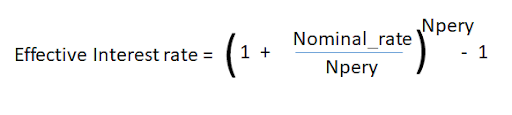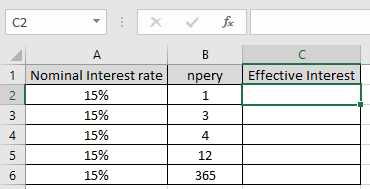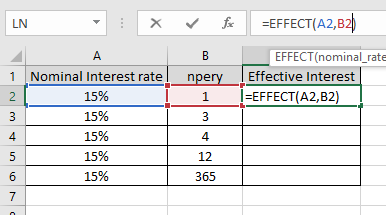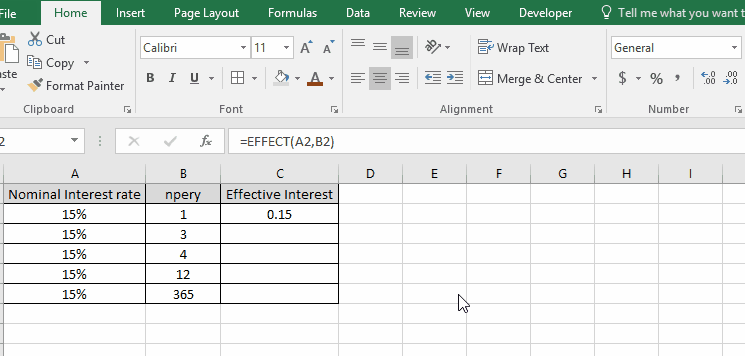# How to use the EFFECT Function in Excel

There are two forms of Interest rate

1. Nominal Interest rate : The nominal interest rate doesn’t take into account the annual compounding period.
2. Effective Interest rate : The effective Interest rate does take into account the annual compounding period and thus returns the accurate measures of Interest charges.

The relation between the two is shown below.Here Npery is number of compounding periods per year

The EFFECT function returns the effective interest rate taking into account the compounding period. It takes nominal_rate & compounding period as arguments.

Syntax:

=EFFECT (nominal_rate, npery)

nominal rate : argument in as percentage or in decimals.
npery : number of compounding period per year

Let’s understand this function using it in an example.

Here we have some Nominal Interest rate values with different compounding periods per year.Use the formula to calculate the Effective Interest rate

=EFFECT (A2, B2)

A2 : Nominal_rate
B2 : compounding period per yearArguments to the formula are taken as cell references.

Change the cell format to percentage.As you can see the required effective Interest rate is in percentage format.

Now copy the formula to other cells using Ctrl + D shortcut key.This is how compounding period changes the Effective Interest rate.

Notes:

1. The function generates #VALUE! Error if arguments to the function is non - numeric.
2. The function generates #NUM! Error if:
• The value of nominal_rate is less than or equal to 0.
• The value of npery is less than one.

Hope you understood how to use EFFECT function and referring cell in Excel. Explore more articles on Excel function here. Please feel free to state your query or feedback for the above article.

Related Articles:

How to Use RATE Function in Excel

How to use the Excel XIRR function

How to Use IRR function in Excel

How to Use NPV function in Excel

Popular Articles:

50 Excel Shortcuts to Increase Your Productivity

How to use the VLOOKUP Function in Excel

How to use the COUNTIF in Excel 2016

How to use the SUMIF Function in Excel

Terms and Conditions of use

The applications/code on this site are distributed as is and without warranties or liability. In no event shall the owner of the copyrights, or the authors of the applications/code be liable for any loss of profit, any problems or any damage resulting from the use or evaluation of the applications/code.